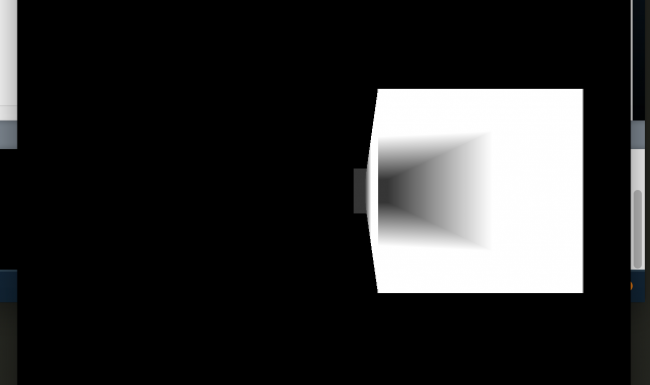#### Howdy, Stranger!

We are about to switch to a new forum software. Until then we have removed the registration on this forum.

# How do I get depth data ?

edited June 2017

I am to try out depth of field and I am first trying to get depth data with GLSL. I am able to generate DEPTH map but there are some glitches I don't understand why?above images shows a long box and it just shows hollow from front face.

this is processing code

``````PShader depth;
PGraphics pg;
void setup()
{
size(600,600,P3D);
pg= createGraphics(600,600,P3D);
depth.set("far",100.0);
}

float t=0;

void draw()
{
t+=0.001;
pg.hint(ENABLE_DEPTH_TEST);
pg.beginDraw();
pg.noStroke();
pg.background(0);
pg.translate(width/2+50,height/2,0);
pg.rotateY(2*PI-PI*t/7);
pg.box(20,20,1000);
//  pg.translate(-200,-100,0);
pg.box(40,40,100);
pg.endDraw();
image(pg,0,0);
}
``````

``````#ifdef GL_ES
precision mediump float;
precision mediump int;
#endif

varying vec4 vertColor;
varying vec3 vertNormal;
varying vec3 vertLightDir;
varying float dist;

void main() {
gl_FragColor = vec4(vec3(1.0,1.0,1.0)*1/clamp(dist,0,1000), 1.0 );
}
``````

``````uniform mat4 transform;
uniform mat3 normalMatrix;
uniform vec3 lightNormal;

attribute vec4 position;
attribute vec4 color;
attribute vec3 normal;

uniform vec3 cameraPosition;
uniform float far;

varying vec4 vertColor;
varying vec3 vertNormal;
varying vec3 vertLightDir;
varying float dist;

void main() {
vertNormal = normal;
dist = (distance(cameraPosition,position.xyz)/far);
gl_Position = transform *position;
}
``````

what could be the issue? well I am just new to GLSL so please sorry for any simple mistake. Also if there is any simple way to do nice DoF let me know.

Tagged:

• to format the code, highlight it and press ctrl-o

• Hey sorry that was the first time. Also I don't think I need to use this pg.hint(ENABLE_DEPTH_TEST);

• https://github.com/edumo/P5PostProcessing here is the code Last week I made it work but did not save it I wounder how it worked then. but it did not gave any good result for blur part. burling was not good.

• edited June 2017

@trailbalzer47 Yes Camera is pointing -Z towards the viewer

to fix the above scetch you need to add as third parameter the negative camera direction

`pg.translate(width/2+50,height/2,0, -100)`

or fix it in the shader.

no backfaceculling per default

https://forum.processing.org/one/topic/how-to-enable-backface-culling-in-opengl-to-speed-3d-model-fps.html

Here you did it right: https://github.com/edumo/P5PostProcessing/blob/master/Dof/Dof.pde#L77

``````//quick fix
//no time to write any notes
PGraphics pg;

void setup(){
size(600,600,P3D);
pg= createGraphics(600,600,P3D);
depth.set("far",100.0);
}
float t=0;

float z = 100;

void draw(){
t+=0.001;
pg.hint(ENABLE_DEPTH_TEST);
pg.beginDraw();
pg.noStroke();
pg.background(0);

//flip sign +/- translate.z 100
if(frameCount%60==0) z*=-1.;

//processing.org/reference/PShape_translate_.html
pg.translate(width/2+50,height/2,z);

pg.rotateY(2*PI-PI*t/7);
pg.box(20,20,1000);
//  pg.translate(-200,-100,0);
pg.box(40,40,100);
pg.endDraw();
image(pg,0,0);
}
``````
• I understood it was my mistake simple explanation is that box was too big in Z direction and thus camera went inside the box as it rotated so after reducing size of box the problem is solved its nothing to do with the shader is the camera position intersecting the shape. and thanks to @nabr and @koogs

• edited June 2017 Answer ✓

Ah, yes,
the second box is set to 1000, it looks like their is a face missing, i thought, it has something to do with backfaceculling .

#### Great!

``````PShader depth;
PGraphics pg;
void setup(){
size(600,600,P3D);
pg= createGraphics(600,600,P3D);
depth.set("far",100.0);
}
float t=0;
void draw(){
t+=0.001;
pg.hint(ENABLE_DEPTH_TEST);
pg.beginDraw();
pg.noStroke();
pg.background(0);
pg.translate(width/2+50,height/2,0);
pg.rotateY(2*PI-PI*t/PI);

//changed
pg.box(20,20,936.09999);

pg.box(40,40,100);

pg.endDraw();
image(pg,0,0);
}
``````